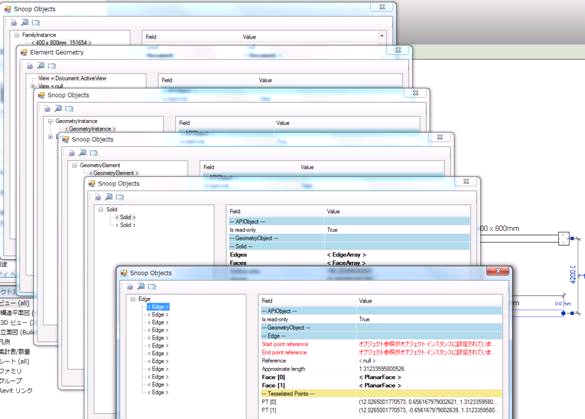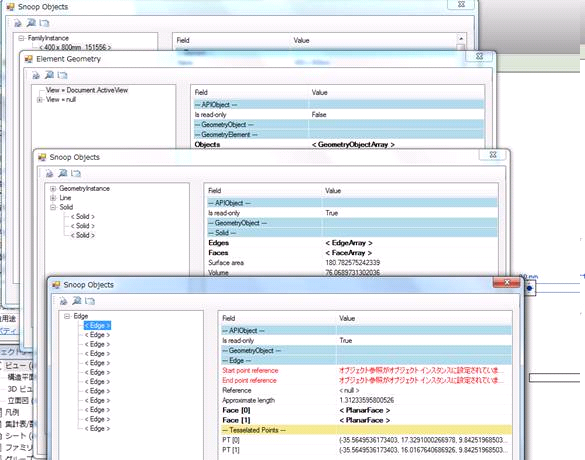### Joined Beam Geometry Access

We have already discussed situations where an element's geometry needs to be accessed in various ways depending on the circumstances, such as the retrieval of unmodified element geometry or the different approaches required to access column and stair geometry, depending on whether the element has its own solid definition or is reusing the solid defined by the symbol it references. Here is a similar solution to obtain the geometry of a beam joined with columns from my colleagues Katsuaki Takamizawa and Harry Mattison:

Question: The NewRebar SDK sample works well with a standalone beam and can access the edges of the beam geometry using the following sequence of calls, illustrated using RevitLookup:However, it rejects a beam joined with columns because it cannot access its edges. In this case, the edges can be obtained differently like this:Should the access to edges (and geometry in general) be implemented differently depending on whether a beam is joined or not? We need to find the beam coordinates to add rebars to it.

Answer: I am unable to reproduce the problem you describe.

The following code used with a specific file finds the same number of edges for both the joined and non-joined beams.

```[Transaction( TransactionMode.Automatic )]
[Regeneration( RegenerationOption.Manual )]
public class CmdGetColumnGeometry : IExternalCommand
{
public Result Execute(
ExternalCommandData commandData,
ref string message,
ElementSet elements )
{
Document doc = commandData.Application
.ActiveUIDocument.Document;

Options options = new Options();

options.ComputeReferences = true;

GeometryElement geomElement = doc
.Selection.PickObject( ObjectType.Element )
.Element.get_Geometry( options );

int edgeCount = 0;
foreach( GeometryObject geomObj
in geomElement.Objects )
{
if( geomObj is GeometryInstance )
{
GeometryInstance inst = geomObj
as GeometryInstance;

if( inst != null )
{
GeometryElement geomElem
= inst.GetSymbolGeometry();

foreach( Object o in geomElem.Objects )
{
Solid solid = o as Solid;
if( solid != null )
{
foreach( Face face in solid.Faces )
{
foreach( EdgeArray edgeArray
in face.EdgeLoops )
{
edgeCount += edgeArray.Size;
}
}
}
}
}
}
}
TaskDialog.Show( "Revit", "Edges: "
+ edgeCount.ToString() );

return Result.Succeeded;
}
}
```

Response: The problem seems to be specific to concrete beams. The code above did not find the geometry objects of a concrete beam joined with columns.

Answer: You are right in that, depending on the specific element and condition, the GeometryElement may contain the desired geometry as a Solid or GeometryInstance. The following code handles both of these cases, as described in Chapter 20 of the Developer Guide:

```  Document doc = commandData.Application
.ActiveUIDocument.Document;

Options options = new Options();
options.ComputeReferences = true;

GeometryElement geomElement = doc.Selection
.PickObject( ObjectType.Element )
.Element.get_Geometry( options );

int ctr = 0;
foreach( GeometryObject geomObj
in geomElement.Objects )
{
if( geomObj is Solid )
{
FaceArray faces = ( ( Solid ) geomObj ).Faces;
ctr += faces.Size;
}

if( geomObj is GeometryInstance )
{
GeometryInstance inst = geomObj
as GeometryInstance;

if( inst != null )
{
GeometryElement geomElem
= inst.GetSymbolGeometry();

foreach( Object o in geomElem.Objects )
{
Solid solid = o as Solid;
if( solid != null )
{
ctr += solid.Faces.Size;
}
}
}
}
}
TaskDialog.Show( "Revit", "Faces: " + ctr );
}
```

Response: Thank you, your code above and also the code in Chapter 20.5 of the developer guide worked for the concrete beam.